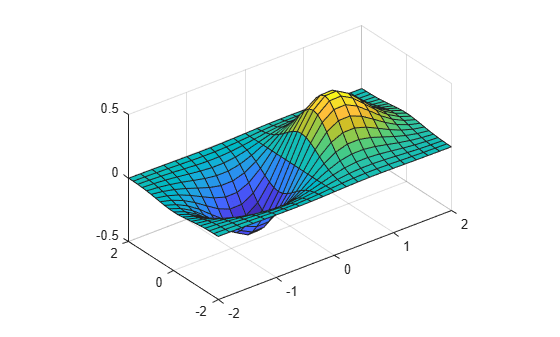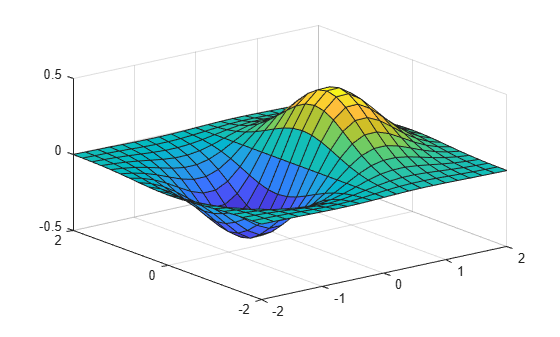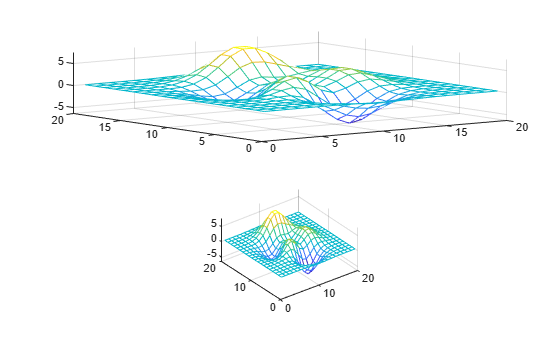Documentation

# pbaspect

Control relative lengths of each axis

## Syntax

``pbaspect(ratio)``
``pb = pbaspect``
``pbaspect auto``
``pbaspect manual``
``m = pbaspect('mode')``
``___ = pbaspect(ax,___)``

## Description

example

````pbaspect(ratio)` sets the plot box aspect ratio for the current axes. The plot box aspect ratio is the relative length of the x-axis, y-axis, and z-axis. Specify `ratio` as a three-element vector of positive values that represent the ratio of the x-axis, y-axis, and z-axis lengths. For example, `[3 1 1]` specifies that the length of the x-axis is equal to three times the length of the y-axis and z-axis. For equal axis lengths in all directions, use `[1 1 1]`.```

example

````pb = pbaspect` returns the plot box aspect ratio for the current axes.```

example

````pbaspect auto` sets an automatic mode, enabling the axes to choose the appropriate plot box aspect ratio. The mode must be automatic to enable the “stretch-to-fill” feature of the axes.```
````pbaspect manual` sets a manual mode and uses the ratio stored in the `PlotBoxAspectRatio` property of the `Axes` object. When the mode is manual, it disables the stretch-to-fill feature of the axes. Specifying a value for the plot box aspect ratio sets the mode to manual. ```

example

````m = pbaspect('mode')` returns the current mode, which is either `'auto'` or `'manual'`. By default, the mode is automatic unless you specify the plot box aspect ratio or set the mode to manual.```

example

````___ = pbaspect(ax,___)` uses the axes specified by `ax` instead of the current axes. Specify the axes as the first input argument for any of the previous syntaxes. Use single quotes around the `'auto'` and `'manual'` inputs.```

## Examples

collapse all

Create a simple line plot. Make the x-axis, y-axis, and z-axis (not shown) equal lengths.

```x = linspace(0,10); y = sin(x); plot(x,y) pbaspect([1 1 1])```Plot a surface and make the x-axis twice as long as the y-axis and z-axis.

```[x,y] = meshgrid(-2:.2:2); z = x.*exp(-x.^2 - y.^2); surf(x,y,z) pbaspect([2 1 1])```When you specify the plot box aspect ratio, the associated mode changes to manual. Query the current plot box aspect ratio mode.

`m = pbaspect('mode')`
```m = 'manual' ```

Create a 3-D scatter chart of random data and set the plot box aspect ratio. Then revert back to the default plot box aspect ratio.

```X = rand(100,1); Y = rand(100,1); Z = rand(100,1); scatter3(X,Y,Z) pbaspect([3 2 1])````pbaspect auto`Create a surface plot.

```[x,y] = meshgrid(-2:.2:2); z = x.*exp(-x.^2 - y.^2); surf(x,y,z)```Query the plot box aspect ratio. The values indicate the ratio of the x-axis length to y-axis length to z-axis length.

`pb = pbaspect`
```pb = 1×3 1.0000 0.9411 0.8496 ```

Starting in R2019b, you can display a tiling of plots using the `tiledlayout` and `nexttile` functions. Call the `tiledlayout` function to create a 2-by-1 tiled chart layout. Call the `nexttile` function to create the axes objects `ax1` and `ax2`. Plot into each of the axes. Then set the plot box aspect ratio for lower plot by specifying `ax2` as the first input argument to the `pbaspect` function.

```tiledlayout(2,1) ax1 = nexttile; mesh(peaks(20)) ax2 = nexttile; mesh(peaks(20)) pbaspect(ax2,[2 2 1])```## Input Arguments

collapse all

Plot box aspect ratio, specified as a three-element vector of positive values. For example, `pbaspect([3 2 1])` specifies that the ratio of the x-axis length to y-axis length to z-axis length is 3 to 2 to 1. Thus, the x-axis is the longest and the z-axis is the shortest.

Specifying the ratio sets the `PlotBoxAspectRatio` property for the `Axes` object to the specified value. The `PlotBoxAspectRatio` property interacts with the `DataAspectAspectRatio`, `XLim`, `YLim`, and `ZLim` properties to control the length and scale of the x-axis, y-axis, and z-axis.

Specifying the ratio sets the `PlotBoxAspectRatioMode` property to `'manual'` and disables the stretch-to-fill behavior of the axes.

Data Types: `single` | `double` | `int8` | `int16` | `int32` | `int64` | `uint8` | `uint16` | `uint32` | `uint64`

Target axes, specified as an `Axes` object. If you do not specify the axes, then `pbaspect` uses the current axes (`gca`).

## Output Arguments

collapse all

Current plot box aspect ratio, returned as a three-element vector of values representing the ratio of the x-axis, y-axis, and z-axis lengths.

Querying the plot box aspect ratio returns the `PlotBoxAspectRatio` property value for the `Axes` object.

Current plot box aspect ratio mode, returned as either `'auto'` or `'manual'`. When the mode is automatic, MATLAB® determines the appropriate plot box aspect ratio value. If you specify a value, then the mode changes to manual.

Querying the plot box aspect ratio mode returns the `PlotBoxAspectRatioMode` property value for the `Axes` object.

collapse all

### Stretch-to-Fill

When the “stretch-to-fill” behavior is enabled, MATLAB stretches the axes to fill the available space. The axes might not exactly match the data aspect ratio, plot box aspect ratio, and camera-view angle values stored in its `DataAspectRatio`, `PlotBoxAspectRatio`, and `CameraViewAngle` properties.

If you specify the data aspect ratio, plot box aspect ratio, or camera-view angle, then the “stretch-to-fill” behavior is disabled. When stretch-to-fill is disabled, MATLAB makes the axes as large as possible within the available space and strictly adheres to the property values so that there is no distortion. For more information, see Control Axes Layout.

## Algorithms

The `pbaspect` function sets and queries several axes properties related to the data aspect ratio.